Practice Questions: Chemical Reactions & Equations

# Practice Questions: Chemical Reactions & Equations - Science Class 10

## Fill in the Blanks

Q 1. Addition of hydrogen in a substance in a reaction is known as ______ reaction.

Reduction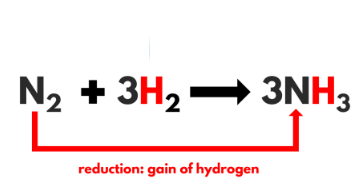Q 2. In a ______ reaction, two or more substances combine to form a new single substance.

Combination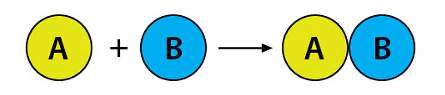Q 3. Unbalanced reactions are also known as ______.

Skeletal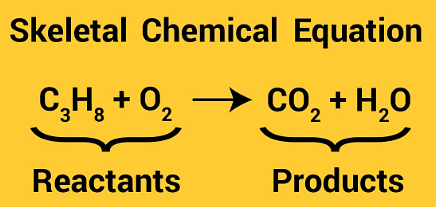Q 4. Reactions in which heat is given out along with the products are called ______ reactions.

Exothermic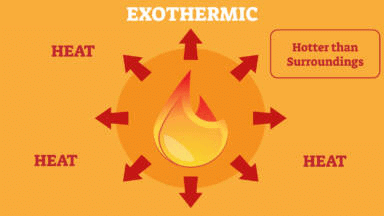Q 5. Reactions in which energy is absorbed are known as ______.

Endothermic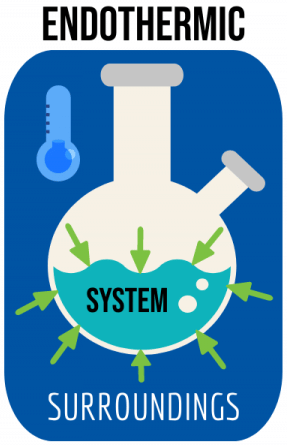Q 6. When an element displaces another element from its compound, a  ______  reaction occurs.

Displacement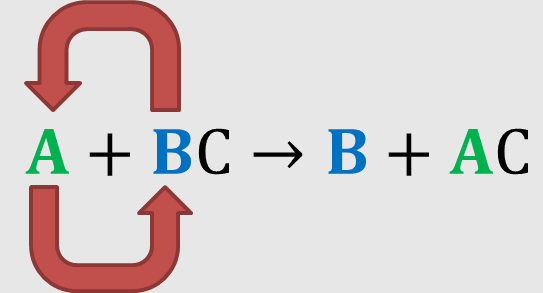Q 7. Those reactions, in which two compounds react by an exchange of ions to form two new compounds, are called ______ reactions.

Double displacement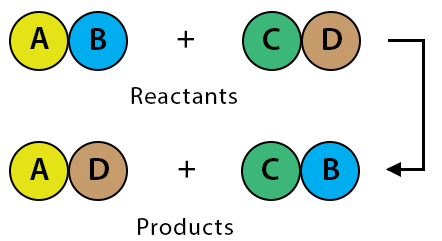Q 8. Precipitation reactions produce ______ salts.

Insoluble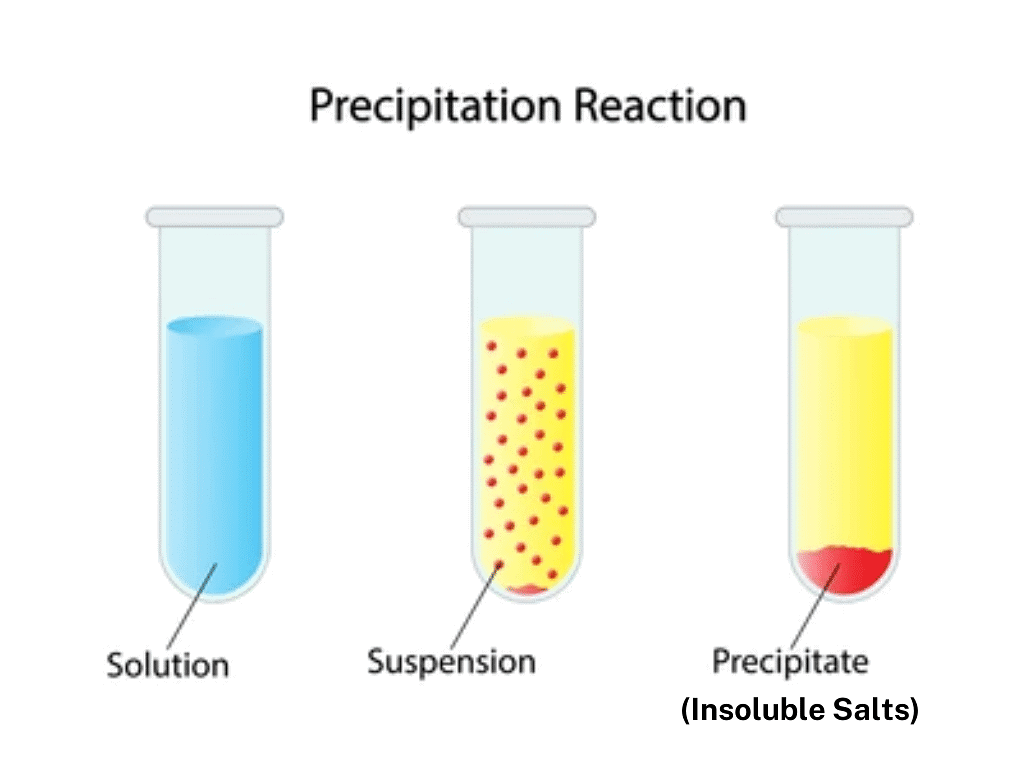Q 9. The reduction is the ______ of oxygen or gain of hydrogen.

Loss

Q 10. The digestion of food in the body is an example of ______ reaction.

Decomposition reaction

Q 11. The addition of oxygen to a substance is called ______.

Oxidation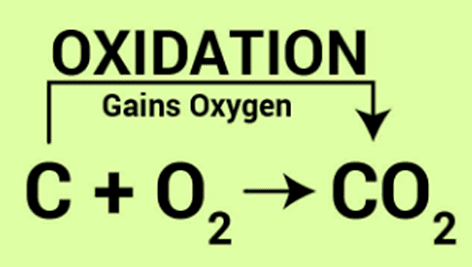Q 12. When calcium carbonate is heated, it decomposes to give ______ and ______.

CaO(s) and CO2 (g)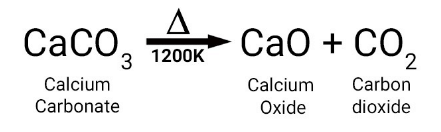Q 13. Complete the missing components/variables given as x and y in the following reactions:
(a) Pb (NO3)2 (aq) + 2KI (aq) → Pbl2 (x) + 2KNO3 (y)

(b) Cu (s) + 2AgNO3 (aq) → Cu (NO3)2 (aq) + x (s)

(a) x → (s); y → (aq)
(b) x → 2Ag

## True or False

Q 1. The number of atoms of each element is conserved in any chemical reaction.

True

Explanation: The law of conservation of mass applies in all chemical equations. This means that the number of atoms of products present is conserved in the number of atoms of reactants.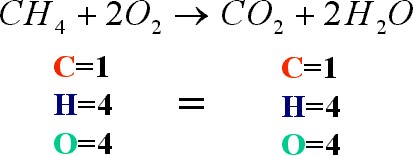Q 2. Oxidation is the loss of electrons from a substance.

True
Explanation: Oxidation is a reaction that removes an electron from a substance.

Q 3. Reduction is the gain of electrons by a substance.

True

Explanation: The gain of electrons is called reduction. Because any loss of electrons by one substance must be accompanied by a gain in electrons by something else, oxidation and reduction always occur together.

Q 4. A complete chemical equation represents the reactants, products and their physical states symbolically.

True

Explanation: A chemical equation is the symbolic representation of a chemical reaction in the form of symbols and formulae, wherein the reactant entities are given on the left-hand side and the product entities on the right-hand side.

Q 5. A magnesium ribbon burns with a dazzling flame in the air (oxygen) and changes into a white substance, magnesium oxide.

True

Explanation: When magnesium ribbon is burnt in the air that is combustion reaction of the magnesium metal. On burning in the presence of oxygen magnesium gives white colour magnesium oxide. Here, magnesium metal is getting oxidized into magnesium ion.

Q 6. Rusting is a double decomposition reaction.

False

Q 7. The reaction between nitrogen and hydrogen to give ammonia is an example of a combination reaction.

True

Explanation: When hydrogen gas combines with nitrogen to form Ammonia.
The following chemical reaction will take place:
Our equilibrium reaction will be N2(g) + 3H2(g) ⇔ 2NH3(g) + Heat.

In this case, Hydrogen and nitrogen react together to form ammonia.

Q 8. The action of heat on ferrous sulphate is an example of a decomposition reaction.

True
Explanation: On heating, ferrous sulphate crystals lose water, and anhydrous ferrous sulphate (FeSO4) is formed. So, their colour changes from light green to white. On further heating, anhydrous ferrous sulphate decomposes to form ferric oxide (Fe2O3), sulphur dioxide (SO2) and sulphur trioxide (SO3).

2FeSO→ ΔFe2O+ SO+ SO3

The reaction for the decomposition of ferrous sulphate is as above.

Q 9. The formation of Na+ and CI- ions from sodium and chlorine is an example of a redox reaction.

True

Explanation: When sodium metal reacts with chlorine gas to form sodium chloride (NaCl), the sodium metal loses an electron, which is then gained by chlorine.

## Very Short Answer Type Questions

Q 1. Which substance is flushed in bags of potato chips to prevent rancidity?

Nitrogen

Q.2. What is the name of reaction in which an atom, molecule or ion gains oxygen?

Oxidation reaction

Q 3. What is the name of reaction in which an atom, molecule or ion loses oxygen?

Reduction reaction

Q 4. What is the chemical name of rust?

Iron oxide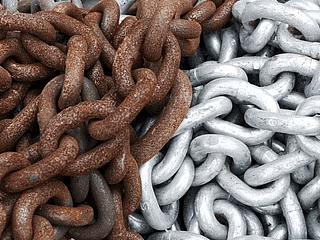Chemical Name of Rust is Iron (III) Oxide

Q 5. Which substance does get oxidized in the rancidity of food?

Fats present in food.

Q 6. In which reaction generally heat is evolved?

Exothermic reaction

Q 7. In which reaction generally heat is absorbed?

Endothermic reaction

Q 8. Which reaction is used in black and white photography?

Thermal decomposition

Q 9. Which chemical substance is used to print the impression of black and white photography paper?

Silver chloride or Silver bromide.

Q 10. What is the chemical name of quick lime?

Calcium oxide

Q 11. What is the chemical name of limestone?

Calcium carbonate

Q 12. What is the chemical name of slaked lime?

Calcium hydroxide

Q 13. Which chemical substance is used in whitewashing?

Calcium oxide

Q 14. What is the chemical equation?

The symbolic representation of a chemical reaction is called a chemical equation.

Q 15. Can a combination reaction be a redox reaction?

Yes, a combination reaction can be a redox reaction.

• In a combination reaction, two elements are combined to make a single product.

2H+ O= 2H2O (water's formula)

Q 16. Write two characteristics associated with rancid food.

Unpleasant smell and unpleasant taste.

The document Practice Questions: Chemical Reactions & Equations | Science Class 10 is a part of the Class 10 Course Science Class 10.
All you need of Class 10 at this link: Class 10

## Science Class 10

78 videos|509 docs|153 tests

## FAQs on Practice Questions: Chemical Reactions & Equations - Science Class 10

 1. What are chemical reactions?Ans. Chemical reactions are the process in which one or more substances transform into new substances with different physical and chemical properties.
 2. What is the difference between physical and chemical changes?Ans. Physical changes are the changes in the physical state of matter without any change in the chemical composition, whereas chemical changes involve the formation of new substances with different chemical properties.
 3. What is the law of conservation of mass?Ans. The law of conservation of mass states that mass can neither be created nor destroyed in a chemical reaction. In other words, the total mass of the reactants is equal to the total mass of the products.
 4. What are the different types of chemical reactions?Ans. The different types of chemical reactions are combination, decomposition, displacement, double displacement, oxidation-reduction, and combustion reactions.
 5. How do you balance a chemical equation?Ans. To balance a chemical equation, the number of atoms of each element should be equal on both sides of the equation. This can be achieved by adjusting the coefficients of the reactants and products.

## Science Class 10

78 videos|509 docs|153 testsExplore Courses for Class 10 examSignup to see your scores go up within 7 days! Learn & Practice with 1000+ FREE Notes, Videos & Tests.
10M+ students study on EduRev
Track your progress, build streaks, highlight & save important lessons and more!
Related Searches

,

,

,

,

,

,

,

,

,

,

,

,

,

,

,

,

,

,

,

,

,

;